Courses
Courses for Kids
Free study material
Free LIVE classes
More

# NCERT Solutions for Class 2 Maths Chapter 4 - Counting In Tens## Perfect Tens: NCERT Solutions for Class 2 Maths Chapter 4 Free PDF Download

Vedantu has subject matter experts from CBSE background who are proficient in solving the problems as per the guidelines from the board. After an extensive research, our experienced teachers and subject experts have formulated the most accurate and appropriated NCERT solutions for Class 2 Maths Chapter 4 as per the guidelines of the Board syllabus. That is why when it comes to the quality then you can be rest assured.

Vedantu offers NCERT Solutions for Class 2 Maths Chapter 4 – Counting in Tens, in the pdf format which is designed as per the latest syllabus issued by (CBSE). NCERT Solutions For Class 2 Maths Chapter 4 Counting in Tens includes all the answers for the questions given in Class 2 Maths Chapter 4 .The subject-experts have also developed reference notes for the same chapter and uploaded on Vedantu official website, which will help you to revise the complete chapter and score good marks in your exams.

If you have any doubts with NCERT Solutions for Class 2 Maths Chapter 4 – Counting in Tens provided by Vedantu then you can also take the help of our teachers.

The concept of skip counting by 10s or tens is very significant. It will help the students in future when they start learning addition. Counting becomes simpler if done in groups of tens.

Last updated date: 19th Sep 2023
Total views: 461.1k
Views today: 7.61k

## Access NCERT Solutions for Class 2 Maths Chapter 4 – Counting in tens

1. In the morning, she counted her chickens.

1. How many baskets of 10 chickens are there? ____________

2. How many chickens are there in all? $50 + 4 =$____________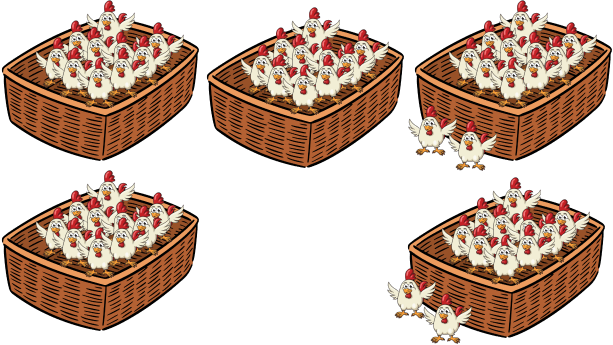Ans:

1. There are 5 baskets, each having 10 chickens.

2. There are a total of 50 chickens in 5 baskets and four outside. $50 + 4 = 54$.

Thus, there are 54 chickens.

2. In the evening, she counted the chickens again.

1. There are ___________ baskets of 10 chickens.

2. There are __________ $+ 3$ = __________ chickens in all.

3. $54{\mkern 1mu} -$ ___________ = ___________ chickens have been eaten by the fox.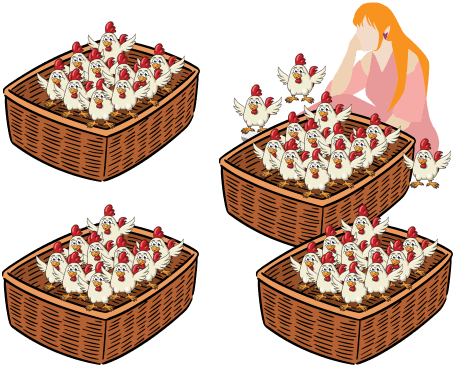Ans:

a) There are 4 baskets of 10 chickens.

b) There are $40 + 3 = 43$ chickens in all.

c) $54 - 43 = 11$ chickens have been eaten by the fox.

How many are These

3. He uses 10 sticks to make 1 bundle.

1. 3 bundles have __________ sticks.

2. Now, how many sticks in all are these? ____________ sticks in all.

3. 4 bundles would have _____________ sticks.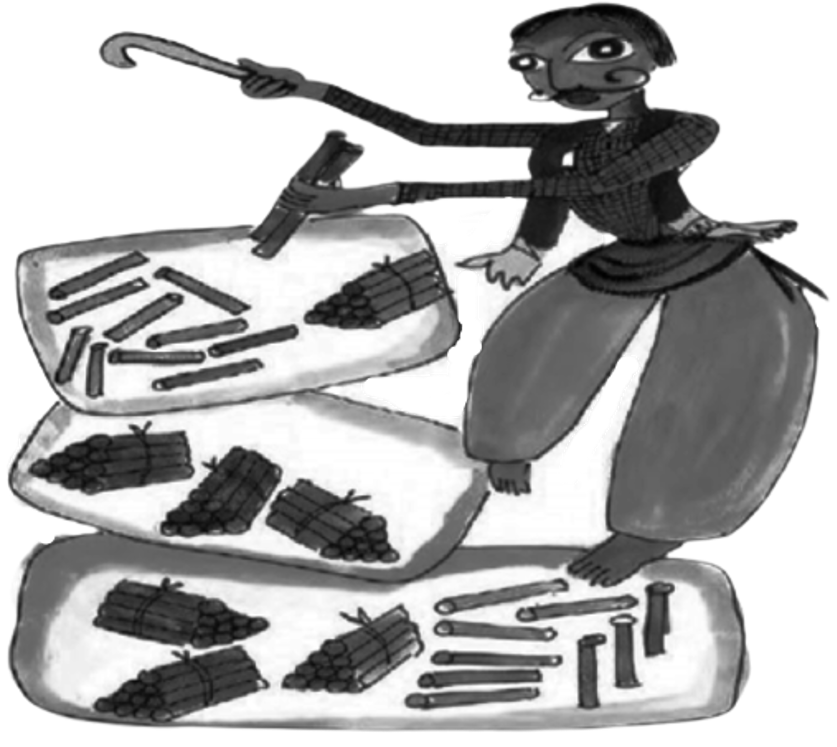Ans:

1. 3 bundles have 30 sticks.

2. 39 sticks in all.

3. 4 bundles would have 40 sticks.

There is a wedding in Malti’s house. She is making flower garlands.4. So there are _______ flowers in all.

Ans: 58

5. How many flowers are these?

Ans: 67 flowers in all.

6. How many garlands of 10 flowers each can you make using 21 flowers? Draw them in the space below.

Ans: If each garland is of 10 flowers, then we can make 2 garlands out of 21 flowers and 1 will be remaining.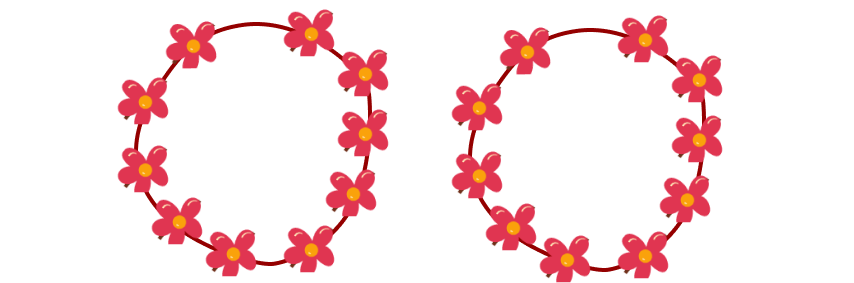7. Let us help Leela.1. How many packets of 10 pencils are there? ____________

2. How many pencils are outside the packets? ____________

3. So, altogether, there are ___________ pencils.

Ans:

1. There are 6 packets of pencils.

2. There are 4 pencils outside the packets.

3. So, altogether,

$\Rightarrow (6 \times 10) + 4 = 64$

There are 64 pencils.

8. Kanak likes collecting different kinds of bindis.

1. How many packets does she have? ___________

2. So how many bindis in all? ___________

(Each packet has $3 + 4 + 3$ bindis.)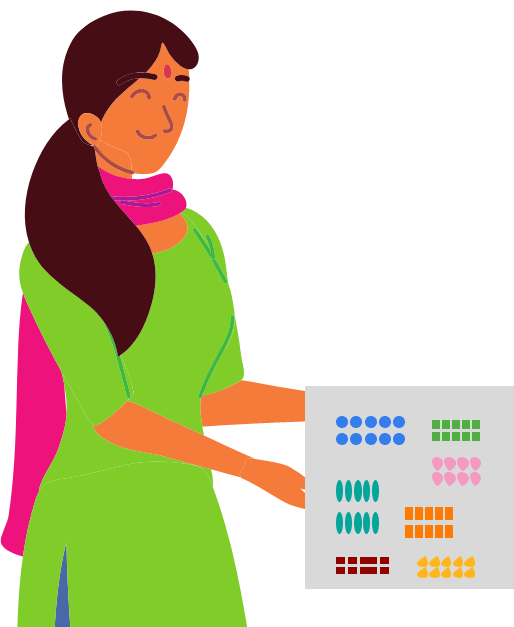Ans:

1. She has 7 packets of bindis.

2. Since each packet has 10 bindis, then there are 70 bindis in all.

## NCERT Solutions for Class 2 Maths Chapter 4 Counting in Tens

### What is Counting by Tens With Numbers?

Counting by Tens with numbers means 10, 20, 30, 40, 50, 60, 70, 80, 90 and so on. So what are we doing here? We are jumping each number by 10 numbers. This is called counting in tens.

You will notice that when we are counting the numbers by 10, we are actually creating a pattern. Have you seen all the numbers are ending in a zero? The first digits are the same as numbers when you count (1, 2, 3, 4, 5, etc.) and the last digit is the same for all the numbers i.e. 0.

### Let Us Understand Better With Examples.

We take a bunch of grapes that have 10 grapes in it. Now we add another bunch of 10 grapes to the first bunch.

What is the total number of grapes now? 10 + 10 = 20 grapes. We add another bunch of 10 grapes to 20 grapes. How many grapes are there in total?

20 + 10 = 30 grapes

In the above example, we are adding 10 grapes each time and jumping to the next tenth number.

Another example: We have 4 bundles of pencils. Out of those 4 bundles, 3 bundles have 10 pencils each and the fourth bundle has 5 pencils.

So how many pencils are there? Let us count by bundles.

10 +        10 +   10  +   5     =      35 pencils

1st bundle           2nd bundle        3rd bundle          4th bundle

### Grouping

Let us understand grouping now.

Example: We have 24 stars. Let us group the stars in tens. How many groups can we make out of 24 stars?

1st group + 2nd group

10   10

We are left with 4 stars. We can make only two groups of stars in tens.

We have 4 stars left. How many stars do we need to make it a group of ten? We need 6 more stars to make it 10. We are adding 6 + 4 = 10.

## What are the Benefits of Solving Ncert Solutions for Class 2 Maths Chapter 4 - Counting in Tens?

Solving NCERT Solutions for Class 2 Maths Chapter 4 - "Counting In Tens" offers several benefits for young learners:

• Mastering Counting Skills: By practicing counting in groups of tens, students develop a strong foundation in basic numerical skills, which is essential for further mathematical concepts.

• Understanding Multiples of Ten: Students grasp the concept of multiples of ten and learn to identify and count numbers efficiently, enhancing their number sense.

• Improved Problem-Solving Abilities: The exercises in the solutions promote critical thinking and problem-solving skills as students encounter different scenarios requiring counting in tens.

• Confidence Building: Successfully solving the problems in the NCERT Solutions boosts students' confidence, encouraging them to tackle more complex math concepts.

• Interactive Learning: The solutions offer interactive and engaging content, making the learning process enjoyable and increasing student engagement.

• Self-Assessment and Revision: Students can self-assess their progress by solving the solutions, identifying areas that require more practice and revision.

• Exam Preparation: Practicing these solutions prepares students for exams as they become familiar with the types of questions that may appear in assessments.

• Application in Real-Life: Counting in tens is a practical skill used in various daily-life situations, such as counting money or objects, and these solutions help students apply this knowledge practically.

### Conclusion

NCERT Solutions for Class 2 Maths Chapter 4 - "Counting In Tens" present an exciting and engaging approach to help young learners master the concept of counting in groups of tens. These solutions offer a structured and comprehensive learning experience, enabling students to confidently count and comprehend numbers in multiples of ten. Through a variety of interactive exercises and activities, children enhance their numerical skills, laying a strong foundation for further mathematical concepts. The user-friendly and child-centric nature of these solutions fosters a positive attitude towards math, making the learning process enjoyable and fruitful. NCERT Solutions play a pivotal role in instilling essential mathematical abilities in students at an early stage, empowering them to excel in their academic journey.

## FAQs on NCERT Solutions for Class 2 Maths Chapter 4 - Counting In Tens

1. What do you understand by counting in tens?

Counting by Tens with numbers means 10, 20, 30, 40, 50, 60, 70, 80, 90 and so on. We are jumping each number by 10 numbers. This is called counting in tens.

2. Why should I opt for Vedantu’s NCERT Solutions?

The NCERT solutions are tailor-made by the subject matter experts and experienced teachers. Vedantu provides updated and comprehensive facts about the subject as per the guidelines of the CBSE board that will help children to understand the concepts better.

3. How do you avail the study materials for NCERT Solutions for Class 2 Maths?

NCERT Solutions for Class 2 Maths and its reference notes per topic are available on Vedantu’s official website. They are available in pdf format and you can download them on your phone, computers, laptop and any other device. The teachers have developed the NCERT Solutions Maths in step-to-step method and they are self-explanatory. The students can revise and solve many questions to master the topic.

4. Why is Vedantu the best way to learn from home?

Vedantu provides a holistic solution to home learning. You can have access to a wide range of questions, notes, references and videos on the official website of Vedantu. Each subject has ample worksheets and mock tests to practice. Our very expert teachers design these NCERT solutions and notes as per your learning style. You can learn or revise them at your own pace and you can focus on key areas of improvement. You will gain a complete understanding of complex concepts therefore develop your confidence for exams.

5. Is Chapter 4 of NCERT Class 2 Maths easy?

Chapter 4 of NCERT Class 2 Maths is easy. It is again a chapter that teaches the bare minimum basics to the students. These basics will help the students learn more advanced concepts in later classes. However, it is also a very important chapter, which means, students must dedicate some time towards this chapter to ensure they do well in their later classes. For more information on this chapter, one can always visit Vedantu’s official website.

6. What are the topics covered in Chapter 4 of NCERT Class 2 Maths?

The topics covered in Chapter 4 of NCERT Class 2 Maths are basically everything related to the number 10. This chapter teaches you how to count in terms of 10.  Vedantu offers comprehensive solutions free of cost. You can also download the solutions if you want. These solutions are available at free of cost on Vedantu website(vedantu.com) and mobile app as well.

7. Where can I get solutions for NCERT Maths Chapter 4?

Students can visit the Vedantu website if they are looking for free online solutions for their NCERT Maths Class 2 Chapter 4. Vedantu gives very accurate and the best chapter-wise solutions for all the NCERT subjects. Apart from Chapter 4 too, you will find solutions for all other chapters and subjects here too. These solutions are created by subject matter experts and are reliable since they follow the CBSE guidelines. These solutions are available at free of cost on Vedantu website(vedantu.com) and mobile app as well.

8. How can I study NCERT Maths Chapter 4?

You can study NCERT Maths Chapter 4 in the following ways-

• Read the whole chapter from your NCERT Maths textbook in a thorough manner.

• If you face any doubts while reading the chapter, then you should get your doubts cleared by your teacher.

• Practise solving all the questions in your textbook exercises and more questions.

• Even after learning the chapter, frequent revision is necessary to ensure the concept is not forgotten.

9. What is NCERT Maths Class 2 Maths Chapter 4 all about?

NCERT Maths Class 2 Chapter 4 deals with the number 10. This chapter teaches the students how to count something with reference to the number 10. The chapter is titled “Counting in Tens.” This forms the basics of Mathematics, which is essential to delve deeper into the subject in higher classes. Easy solutions, important questions and revision notes are available on Vedantu to help the student grasp the subject better.